Computer Science

# Introduction to Bit

For the first part of the course we will be using Bit, a character that you will move around a world. Bit is depicted as a triangle:and his world is a grid of squares: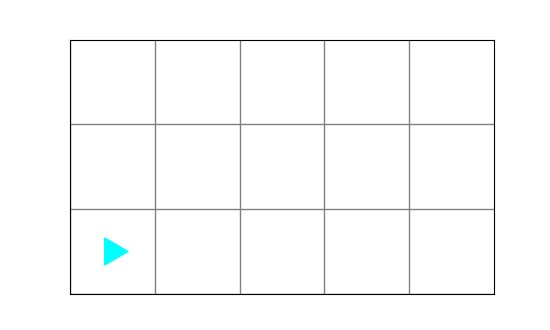Think of Bit as a robot that you control by writing and running code.

## Basic things Bit can do

Some of the things Bit can do are:

• `move` — one square at a time
• `left` — turn left
• `right` — turn right
• `paint` — change the color of a square

To illustrate how Bit works, here is a short Bit program:

``````from byubit import Bit

@Bit.empty_world(5, 3)
def move_around(bit):
bit.move()
bit.move()
bit.paint("red")

bit.left()
bit.move()
bit.paint("green")

bit.right()
bit.move()
bit.paint("blue")

if __name__ == '__main__':
move_around(Bit.new_bit)
``````

We will explain all this code shortly, but for now, focus on these lines:

• `bit.move()` — moves bit one square to the right
• `bit.left()` — turns bit to the left
• `bit.right()` — turns bit to the right
• `bit.paint("red")` — paints the current square red

Each of these commands is a function that you are calling. Every time you call a function you use parentheses: `()`. Inside the parentheses are the arguments or parameters for the function.

The `move()`, `left()` and `right()` functions do not take any arguments. The `paint()` function takes one argument — the name of a color.

Bit understands three colors:

• `"red"`
• `"green"`
• `"blue"`

These must be in double quotes or single quotes.

We can run this program by clicking the green triangle next to the line of code that starts with `if`:We will explain this below. Here is what it looks like in PyCharm: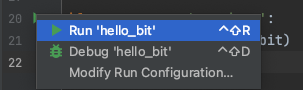Here is what you should see: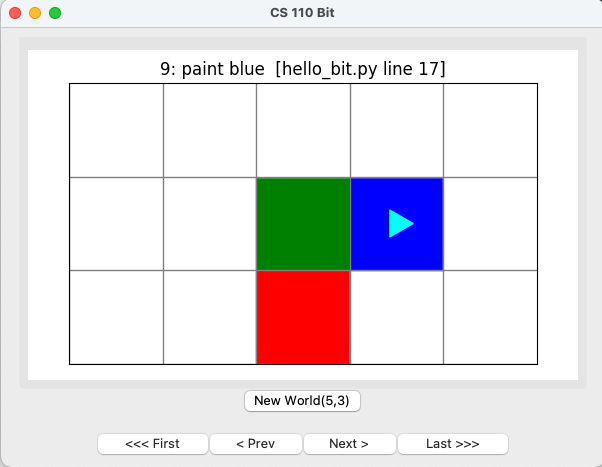This shows that bit moved through the world and painted some squares.

Use these buttons to “replay” what Bit did:

• `First` — moves back to the first line of code
• `Prev` — moves back one line of code
• `Next` — moves forward one line of code
• `Last` — moves to the last line of code

As you move, the top part of the world will tell you which line of code Python is running.

## Running Bit on your own

To get some practice running Bit on your own, create a folder in your `cs110` project called `bit`. You can do this in PyCharm by right-clicking on the `cs110` folder and selecting `New➡️Direectory`:Then create a new file in this folder called `hello_bit.py`. You can do this by right-clicking on the `bit` folder and selecting `New➡️File`: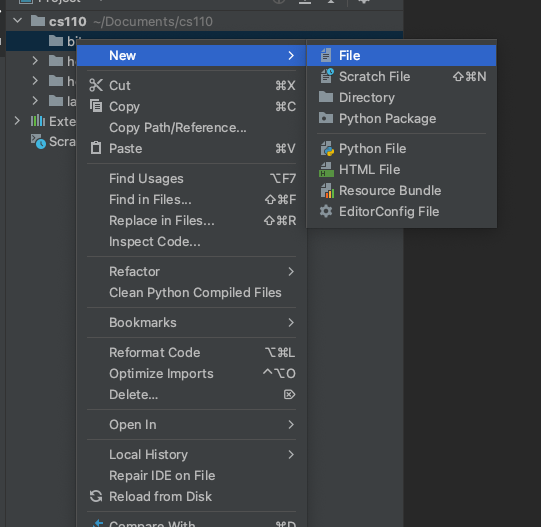Then copy and paste the code above into this file.

You should end up with a file like this:### Installing bit

To run this code, you need to install the `byubit` library. See the Installing Bit guide if you haven’t done this yet.

### Running the program

Now you can run this code on your own. Click the green triangle next to the line of code that starts with `if`:and select `Run 'hello_bit'`. You should see what we showed you above:## Explaining the rest of this code

Let’s go through this code line by line.

### Line 1

Whenever you write a program that uses Bit, you need to start with this line:

``from byubit import Bit``

This is a way of telling Python that you want to use the `byubit` library that you installed. You will use `Bit` with a capital `B` for special commands telling the library what to do.

### Lines 4 to 16

Lines 4 to 16 contain a function called `move_around()`:

``````@Bit.empty_world(5, 3)
def move_around(bit):
bit.move()
bit.move()
bit.paint("red")

bit.left()
bit.move()
bit.paint("green")

bit.right()
bit.move()
bit.paint("blue")``````
• 4: `@Bit.empty_world(5, 3)` — This is a decorator that tells `Bit` to start in an empty world that is `5` squares wide and `3` squaers tall.

• 5: `def move_around(bit):` — This is a function definition. Everything we will do this semester will involve writing Python functions.

• 6 to 16 — This is the function body. The function body will be run every time you call the function.

A function has five parts to it: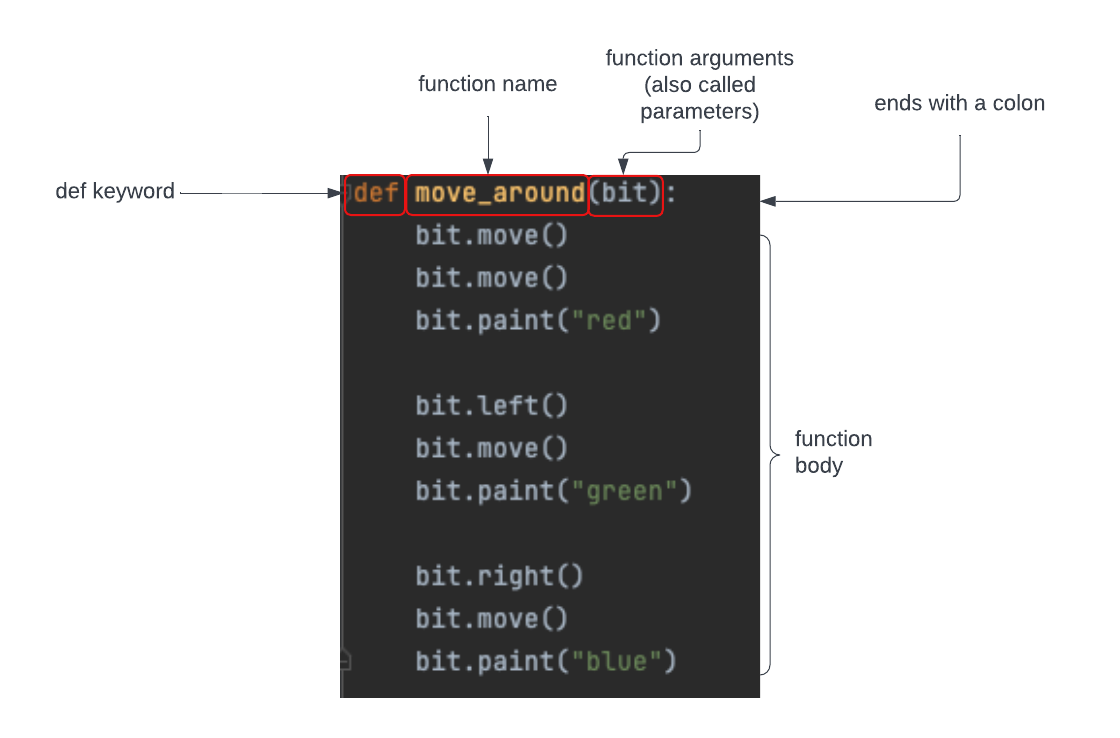1. The `def` keyword tells Python you are defining a function.

2. The function name tells Python how someone can call this function. Here we have given the function the name `move_around()`.

3. The function arguments are listed in parentheses. Here we tell Python this function takes one argument, `(bit)`.

4. The function definition ends with a colon `:`.

5. The function body is the list of commands you will run when the function is called. These are indented and the indentation is very important. Every line in the function body must be indented the same amount. The usual indentation is four spaces.

### Lines 19 and 20

Lines 19 and 20 contain the main block of the Python program:

``````if __name__ == '__main__':
move_around(Bit.new_bit)``````

Every program has to start somewhere. In Python, we call this the main block. This is where Python starts when it runs your code. Python will put a green triangle to the left of your main block so that you can click on it to run your program.

In this main block, we have one line of code:

``move_around(Bit.new_bit)``

Here we are calling the `move_around()` function. We have to give it a bit, and we do this by saying `Bit.new_bit`. This creates a new bit to live in the world.

## Defining versus calling a function

When we define a function with `def`, we are telling Python what a function means. For example, when we defined `move_around()` we told Python this means move Bit two squares, turn left, move another square, paint red, and so forth.

When we call a function, we are telling Python to run all of the code in the function definition. This is when Python will actually run all of the code that tells Bit what to do.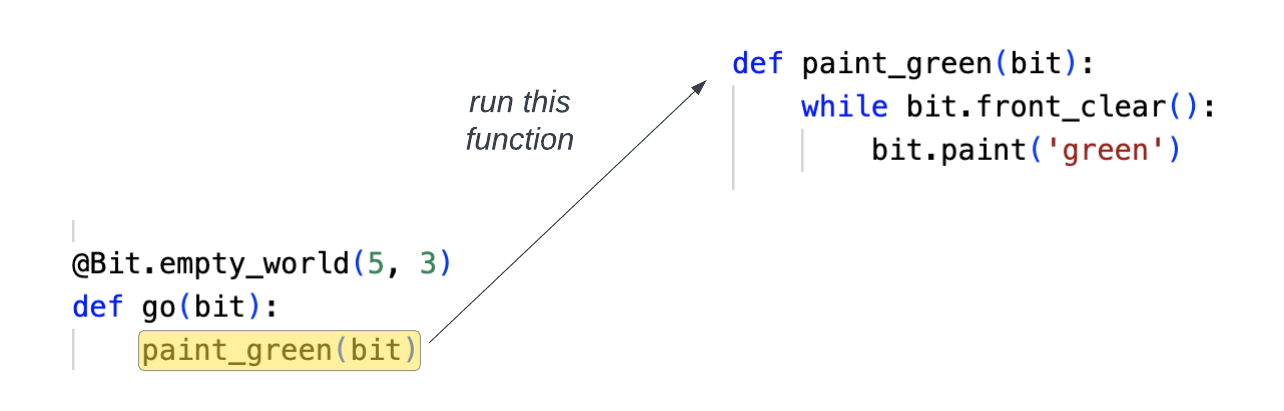## Blank lines

Python needs blank lines to separate pieces of the code. The convention is to use two blank lines between every piece. If you do not follow this convention, PyCharm will complain by putting a squiggly line underneath the line that doesn’t have enough blank lines before it.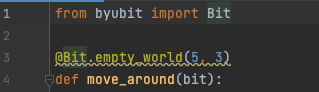Add an extra blank line and the warning will go away.